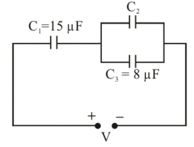In the circuit shown in the figure, the total charge is 750 μC and the voltage across capacitor C2 is 20 V. Then the charge on capacitor C2 is:

# In the circuit shown in the figure, the total charge is $750\text{\hspace{0.17em}}\mathrm{\mu C}$ and the voltage across capacitor ${\mathrm{C}}_{2}$ is 20 V. Then the charge on capacitor C2 is:1. A

$590\text{\hspace{0.17em}}\mathrm{\mu C}$

2. B

$450\text{\hspace{0.17em}}\mathrm{\mu C}$

3. C

$650\text{\hspace{0.17em}}\mathrm{\mu C}$

4. D

$160\text{\hspace{0.17em}}\mathrm{\mu C}$

Register to Get Free Mock Test and Study Material

+91

Verify OTP Code (required)

### Solution:

$\begin{array}{l}\mathrm{Q}={\mathrm{C}}_{1}{\mathrm{V}}_{1}⇒750=15{\mathrm{V}}_{1}⇒{\mathrm{V}}_{1}=50\mathrm{V},{\mathrm{V}}_{2}=20\mathrm{V}\\ {\mathrm{Q}}_{3}={\mathrm{C}}_{3}{\mathrm{V}}_{2}=160\mathrm{\mu c}\\ {\mathrm{Q}}_{2}=750-160=590\mathrm{\mu c}\end{array}$Register to Get Free Mock Test and Study Material

+91

Verify OTP Code (required)